## 3.3 QGIS Field calculator

Now we would like to calculate the building’s rooftop area, the solar radiation each rooftop absorbs, and how much of the solar radiation can be translated into renewable electricity. For these calculations we will use the Field Calculator operator in the attribute table. This calculator enables calculations, based on existing attribute values or defined functions, for instance, to calculate the length or area of geometry features. The results can be used to update an existing field or to create a new field.

### 3.3.1 Building rooftop area

To calculate the building rooftop area, we will open the rosario_municipalb layer’s attribute table and click on the Field Calculator operator. We want to create a new field called area, then we will define the type of the field as a Decimal number. Next, we see the Expression builder log, where we can select which calculation is calculated for that field, we can use the search bar to search for predefined expressions. For our layer, we will use a Geometry expression and calculate the area for the building rooftops, the \$ sign indicates that the calculation is performed on all the buildings in the layer. Under the expression builder log, we can see an Output preview of the values calculated. (See 3.7)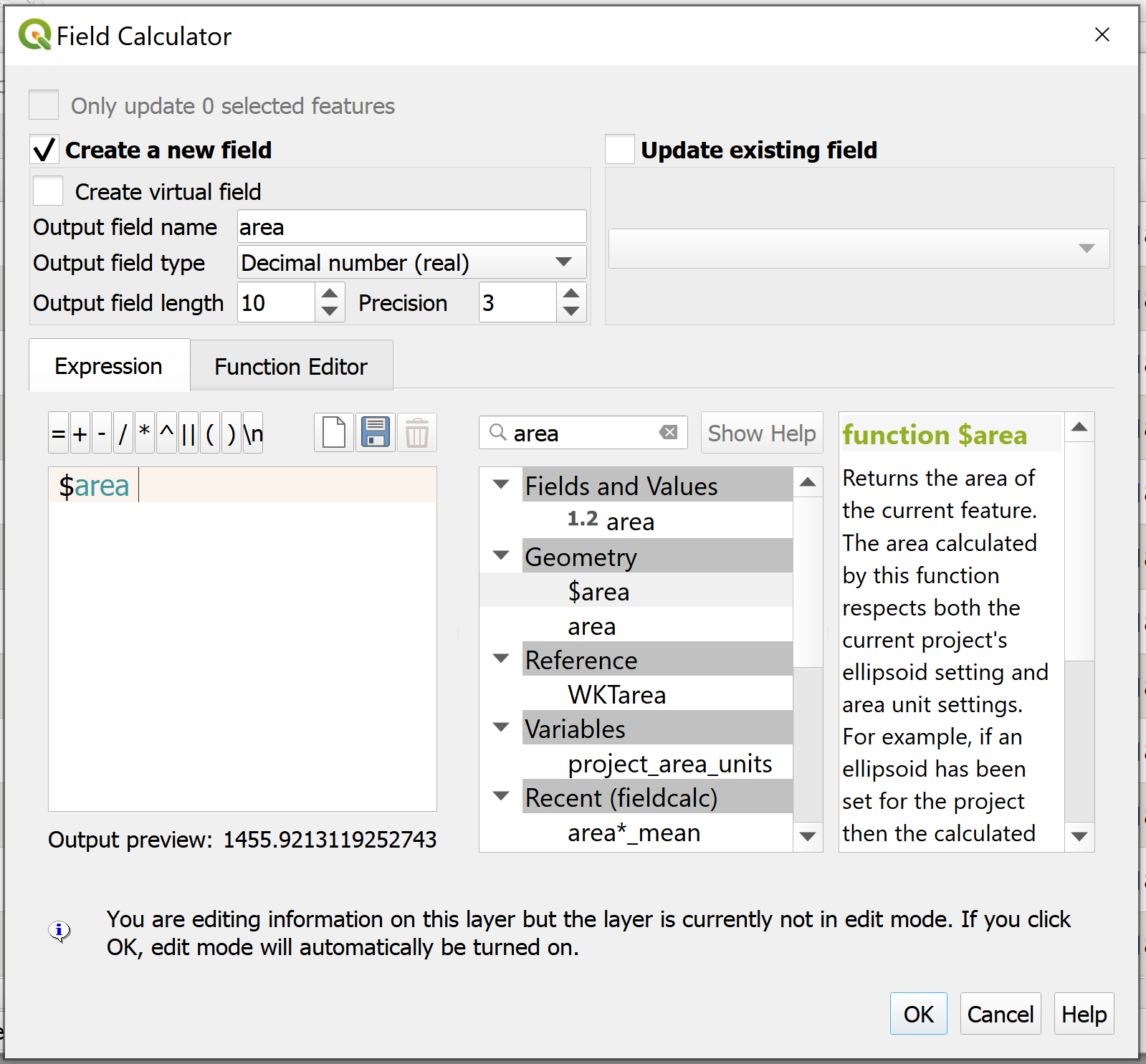Figure 3.7: Field Calculator - Area

Figure 3.8 shows the attribute table of the rosario_municipalb layer with the building rooftop area calculated.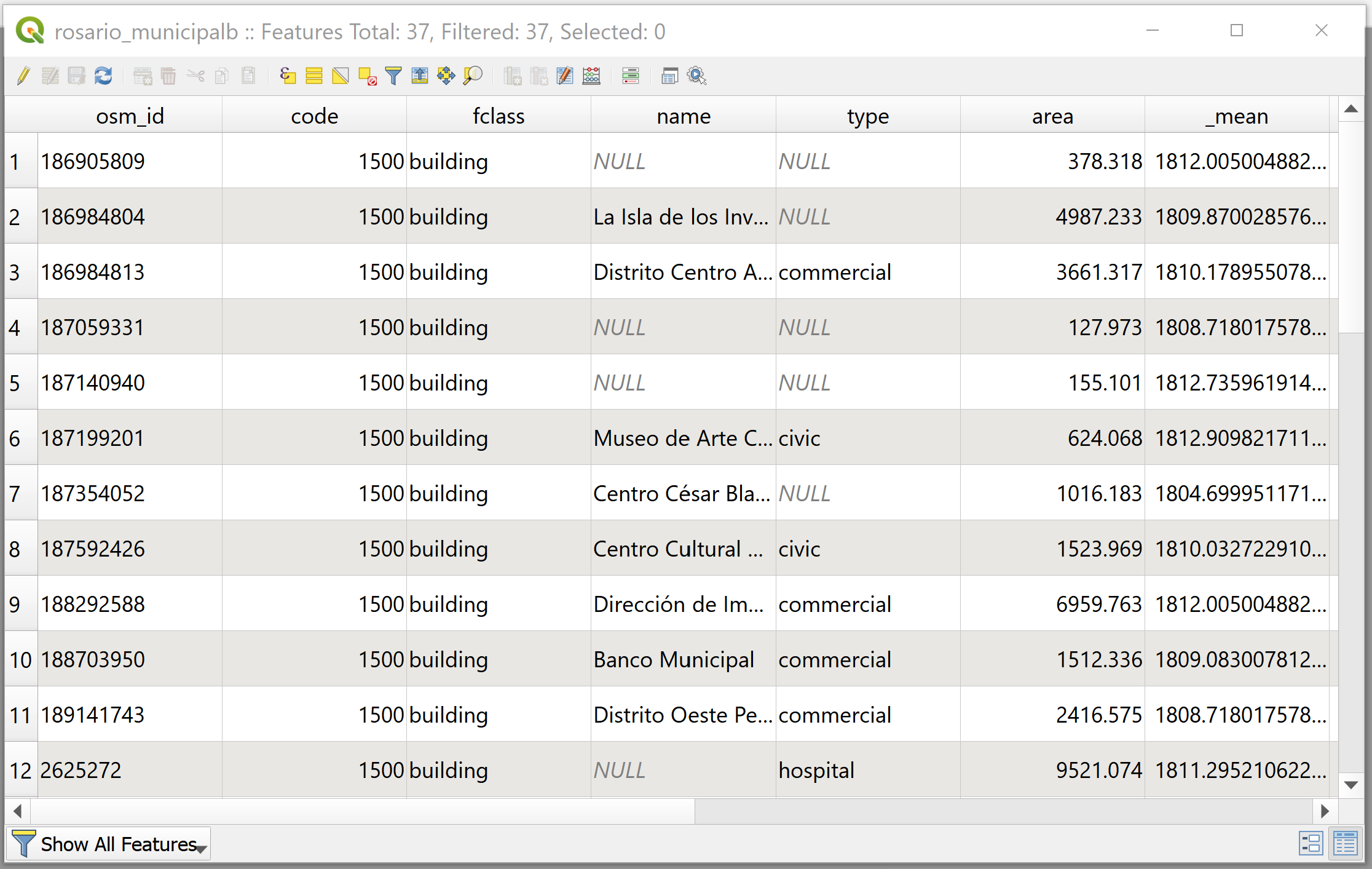Figure 3.8: Attribute Table with Building Rooftop Area Calculation

To calculate the building rooftop solar radiation we will multiply the building rooftop area times the mean GHI value calculated and extracted from the raster layer.

Important! buildings with rooftops under 30 $$m^2$$ are not suitable for solar panel installations.

$\begin{equation} usable\_sr = area * GHI (kWh) \tag{3.1} \end{equation}$

we will open the rosario_municipalb layer’s attribute table and click on the Field Calculator operator. This time, we want to create a new field named usable_sr, then we will define the type of the field as a Decimal number and enter in the Expression builder log our equation (3.1). (See 3.9)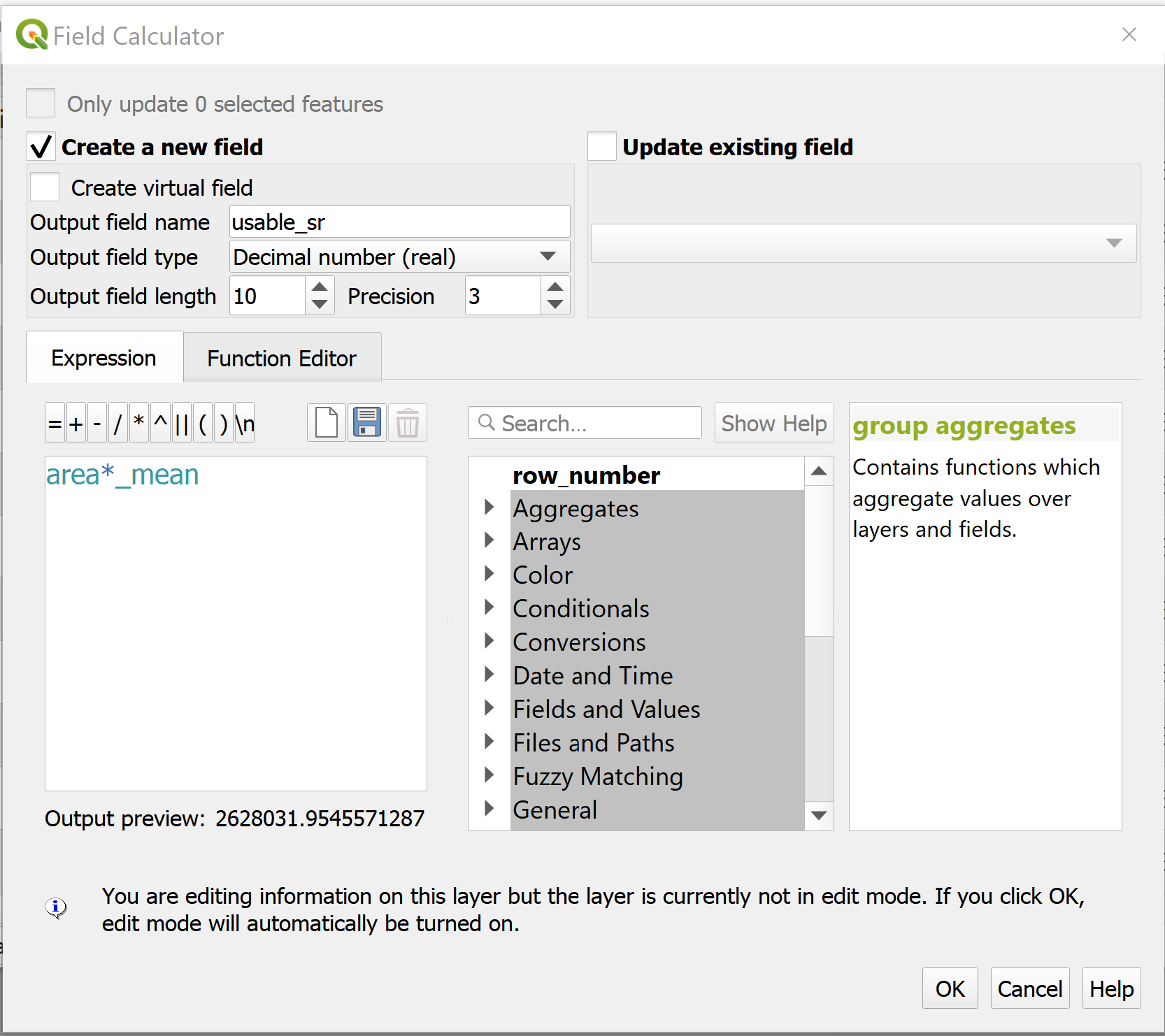Figure 3.9: Field Calculator - Usable Solar Radiation

Figure 3.10 shows the attribute table of the rosario_municipalb layer with the usable solar radiation calculated.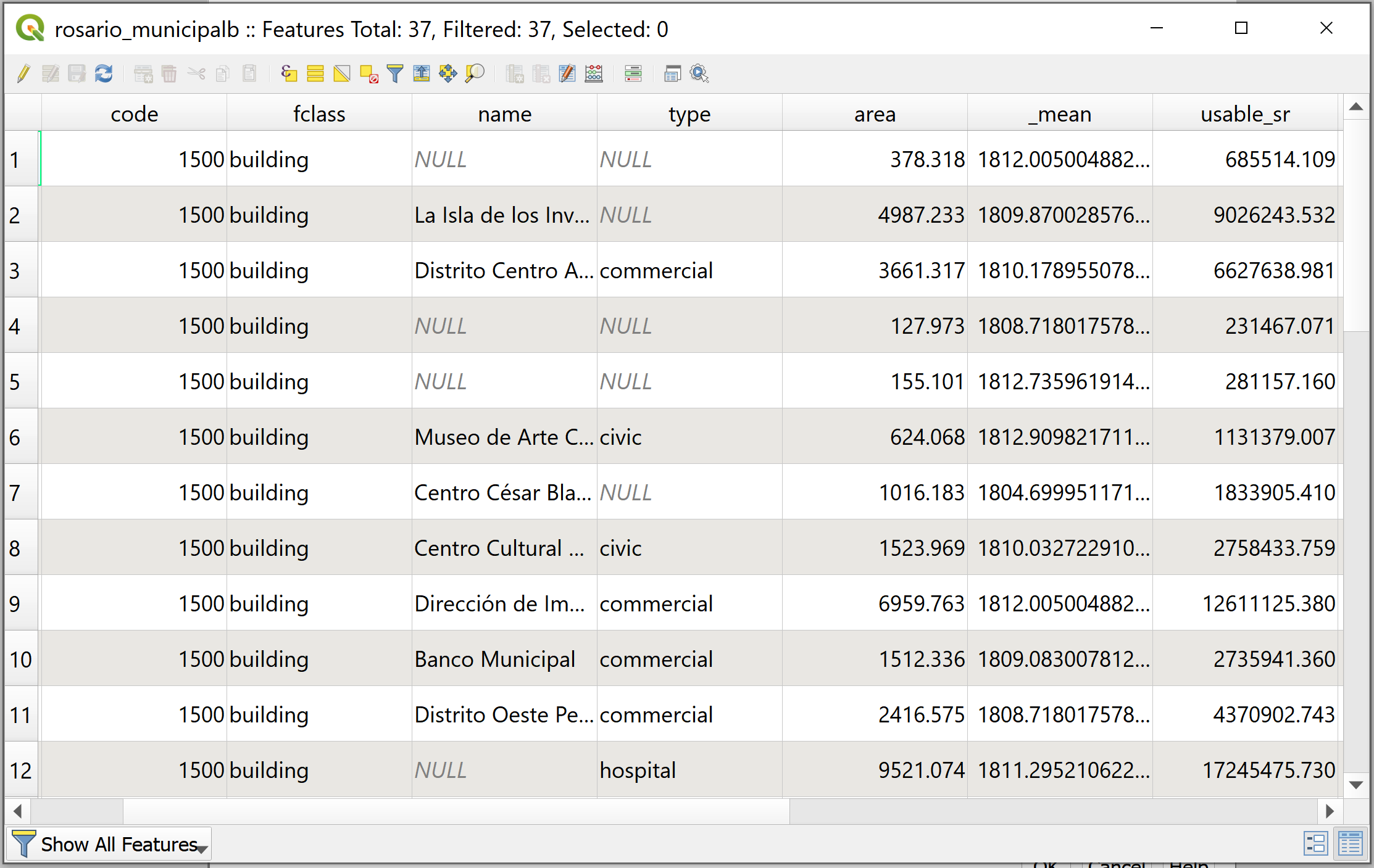Figure 3.10: Attribute Table with Usable Solar Radiation Calculation

### 3.3.3 Renewable electricity production

To calculate the building rooftop renewable electricity production potential we will multiply the usable solar radiation times the solar panel efficiency percentage $$r$$ and a performance ratio percentage $$PR$$. The United States Environmental Protection Agency (EPA) provides the best estimate of 15 percent efficiency and 86 percent performance ratio. These values mean that the solar panels are capable of converting 15 percent of incoming solar energy into electricity, and then 86 percent of that electricity is preserved as it goes through the installation.

$\begin{equation} elec\_prod = usable\_sr * r * PR (kWh) \tag{3.2} \end{equation}$

we will open the rosario_municipalb layer’s attribute table and click on the Field Calculator operator. This time, we want to create a new field named elec_prod, then we will define the type of the field as a Decimal number and enter in the Expression builder log our equation (3.2). (See 3.11)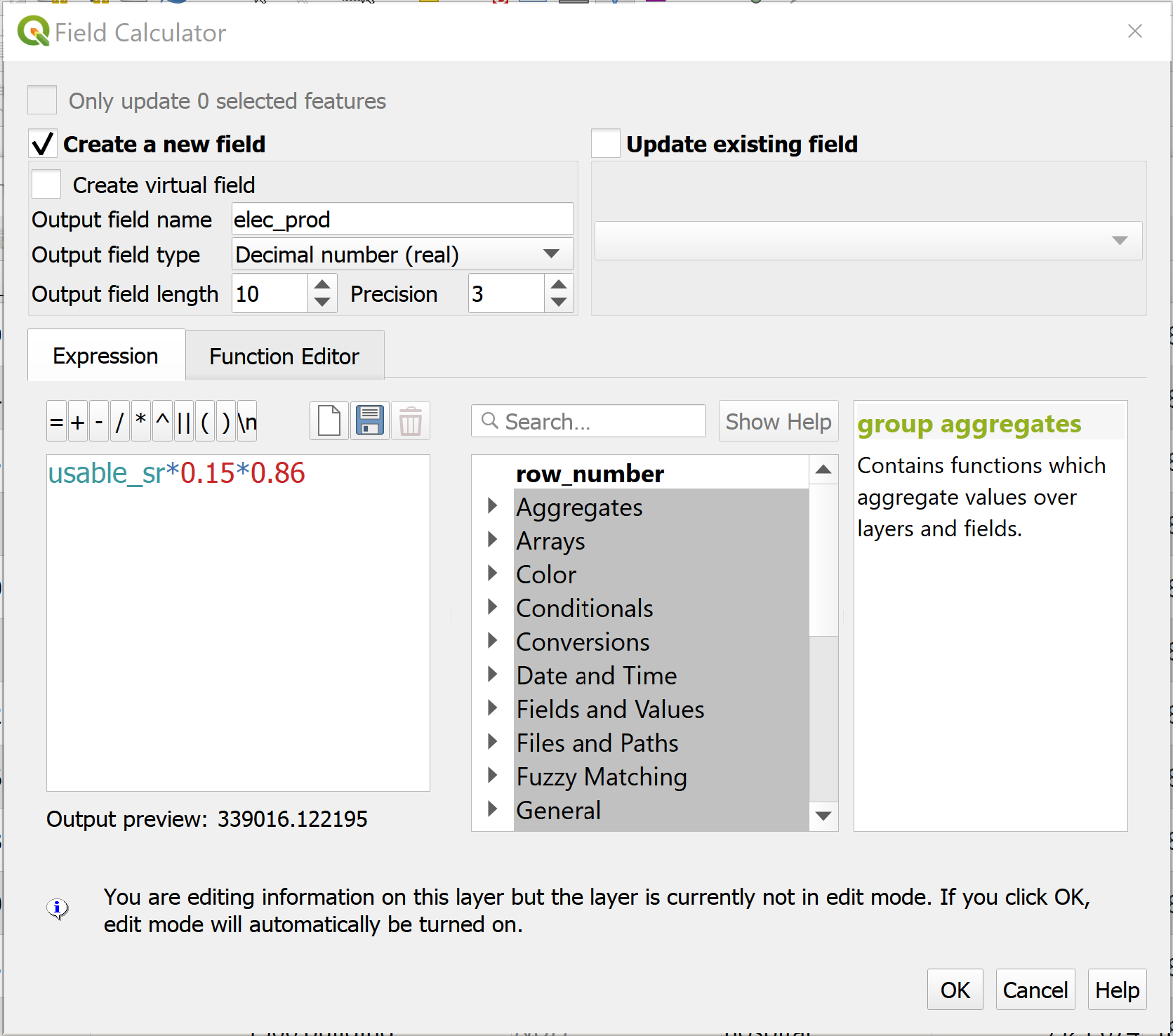Figure 3.11: Field Calculator - Renewable Electricity Production

Figure 3.12 shows the attribute table of the rosario_municipalb layer with the renewable electricity production potential calculated.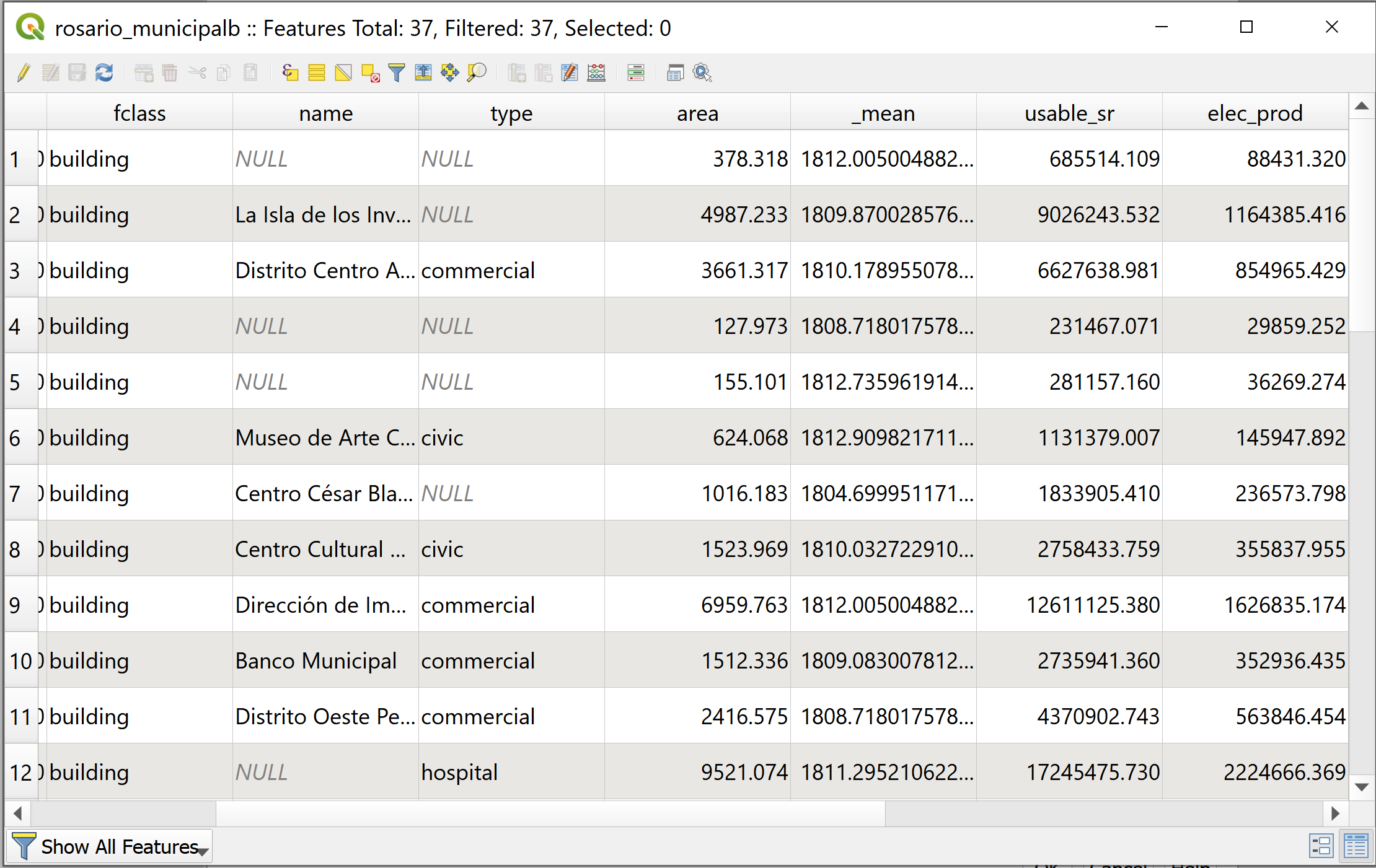Figure 3.12: Attribute Table with Usable Solar Radiation Calculation

As the last step, we can calculate what would be the building’s renewable electricity balance if its rooftop would be equipped with solar panels. For this, we will open once again the Field Calculator operator, create a field named rene_elec and enter the following equation (3.3):

$\begin{equation} rene\_elec = elec\_prod - elec\_consu (kWh) \tag{3.3} \end{equation}$

Where we subtract the building electricity consumed by the renewable electricity production potential. There are 3 possible scenarios for the buildings electricity balance:

1. If the result of the equation is positive $$(+)$$, then the building can supply its own electricity from renewable electric energy and more.
2. If the result of the equation is negative $$(-)$$, then the building can supply a limited amount of renewable electric energy out of the total electric consumption.
3. If the result of the equation is zero $$(0)$$, then the building can supply its electricity from renewable electric energy.

Figure 3.13 shows the attribute table of the rosario_municipalb layer with the renewable electricity balance calculated.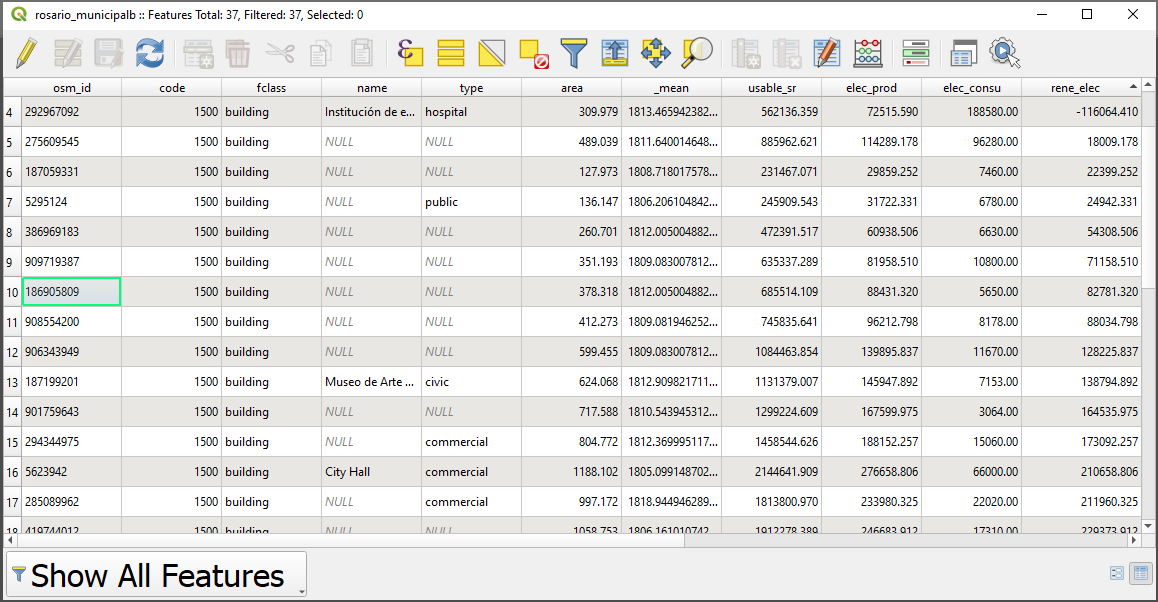Figure 3.13: Attribute Table with Usable Solar Radiation Calculation